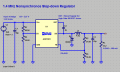# Voltage divider circuit for ESC

#### birdtrick

Joined Dec 1, 2016
21
I'm trying to build my own ESC following a schematic I found online, and something that is tripping me up is the voltage divider circuit. I know it's Electronics 101 stuff but I cannot get the numbers to compute with the different variations I've tried. The circuit image is attached. It is meant to drop the source voltage of 16.8 down to 3.3 for the microcontroller. I'm not getting a number near 3.3 in my math. It's the 4k resistor that I do not know how to place in the equation.

#### jpanhalt

Joined Jan 18, 2008
11,087
Isn't 16.8/5 = 3.4 (approximately)? Put another way, 16.8V/5000Ω = 3.36 mA. 3.36 mA x 1000Ω = 3.36V.

#### birdtrick

Joined Dec 1, 2016
21
But how is the 10k resistor accounted for? I thought it would be (1k+4k)/(10k+4k+1K).

#### jpanhalt

Joined Jan 18, 2008
11,087
1) You fail to show the impedance of VS_Bridge2 to ground. Without that information, I assumed its impedance was very high.
2) Even if that impedance was a dead short, 10K||1K = 0.91 , and 910Ω X3.36mA =3.05V which is "near 3.3V."
3) Your failure to fully define the circuit may be why you fail to understand it.

#### birdtrick

Joined Dec 1, 2016
21
VS_Bridge comes from the FET at the full battery voltage, and this is passed to MUX and needs to be 3.3V. I didn't realize I needed to calculate impedence for the voltage divider. I'm just experimenting with this circuit but will be supplying a 3S source so I'm trying to find the right resistance values. I can just keep swapping them out but would rather understand the equation. It's definitely not as simple as the voltage divider equations one finds from google.#### Deioma87

Joined Feb 17, 2021
2
You can just keep swapping them out but would rather understand the equation. It's definitely not as simple as the voltage divider equations one finds from google.

#### Papabravo

Joined Feb 24, 2006
20,404
Using resistors is a minimally effective sub optimal way to do what you are trying to do. It will only work at a singe value of load current. What you want, although you don't know it yet, is a method that will take a higher voltage in and produce a lower voltage out. It is true that there will be some unavoidable power loss in this process. This can be done fairly easily with circuit boards you can purchase or ones that you design and fabricate your self.

Here is a cheap (less than \$1.00) buck regulator chip with a wide input range.

Here is an example I threw togetherLast edited:

#### Deioma87

Joined Feb 17, 2021
2
An ESC (electronic speed control) is a circuit for controlling an electric motor — particularly brushless DC motors. you can follow GreatScottLab’s great Instructable to build your own robust ESC if you wanted to buy some tools then you can go through the marine equipment wholesaler where you can buy cheap prices. This project just requires an Arduino Nano, an L6234 motor driver, and a handful of common electronic components (resistors, capacitors, etc.)

#### birdtrick

Joined Dec 1, 2016
21

#### crutschow

Joined Mar 14, 2008
32,934
What is the difference between COM_ALL and the circuit ground symbol?

Often when doing voltage divider calculations I find it easier to use node-current equations rather than node-voltage. i.e. sum all the currents into the node to determine the required resistances, since that generally avoids having to write and solve a multi-variable equation.
Thus for the desired voltage of 3.3V at MUX_C, the current through the 1k resistor to ground would be 3.3mA and, if the 4k resistor also goes to ground, its current would be 0.825mA.
The current through R21 must equal that so its value would be (16.8V-3.3V) / 4.125mA = 3.27kΩ.

Last edited:

#### click_here

Joined Sep 22, 2020
548
It sounds like you are having trouble coming up with values for a resistor divider!

I find the best way is to start with the required ratios:
13.5V : 3.3V

Establish the total resistance by giving a current (say 0.5mA), or looking at datasheets for maximum resistance, and then finding close values.

You then look for standard resistor values that are close.

Some close values that I came up with are...
39k:10k,
43k:11k,
47k:12k,
51k:13k

You can change to 5k1:1k3 if you need to keep the input resistance down...

Is this what you are after?